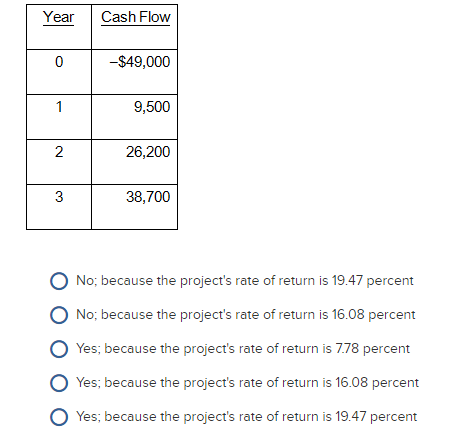### Create an Account

Home / Questions / The Flour Baker is considering a project with the following cash flows Should this project...

# The Flour Baker is considering a project with the following cash flows Should this project be accepted based on its internal rate of return if the required return is 18 percent Year Cash Flow

The Flour Baker is considering a project with the following cash flows. Should this project be accepted based on its internal rate of return if the required return is 18 percent?Year Cash Flow 0 \$49,000 9,500 26,200 a 38,700 O No: because the project's rate of return is 19.47 percent O No because the project's rate of return is 16.08 percent O Yes; because the project's rate of return is 778 percent o Yes: because the project's rate of return is 16.08 percent O Yes because the project's rate of return is 19.47 percent

Apr 06 2020 View more View LessSubscribe To Get Solution